hackernoon.com Go back Open original

# Functional Programming in Python For Beginners | Hacker NoonIn computer science, functional programming is a programming paradigm where programs are constructed by applying and composing functions. (Wikipedia)

The usage of Functional Programming provides us with separation of concern where we can separate data and logic separately. Hence, the code becomes clear and easy to understand to a developer. Functional Programming follows the DRY (Do not Repeat Yourself) principle. Code which follows Functional Programming practice is memory-efficient. The codebase which implements Functional Programming will also be easy to extend and maintain.

## Pure functions

One of the important concepts in functional programming is the usage of pure functions.

A function is said to be a Pure function if:

1. Given the same input, the function will always return the same output.
2. The function must not produce any side effects.

Side effects are things that a function does that affect the outside world, that is they change the state of the program. Changing the data in a variable, printing output can be considered as some examples of side effects of a function.

Consider the following simple example:The function square will always return only the square of a given number and will not change anything in the outside world. This type of functions are also called declarative functions

Note:

But, technically it’s not possible to use pure functions everywhere as we may need to change the state of the code. Although, it’s a good practice to use pure functions as many places as possible. And the fact here is that it highly probable to face bugs and errors occur in non-pure functions rather than in pure functions. Python provides us with some useful pure functions which are built-in python.

## 1) map()

map() accepts two arguments - a function and an iterable.

Consider the above example of square function, we can make use of map() function to quickly apply the function to a given iterable like a list of numbers: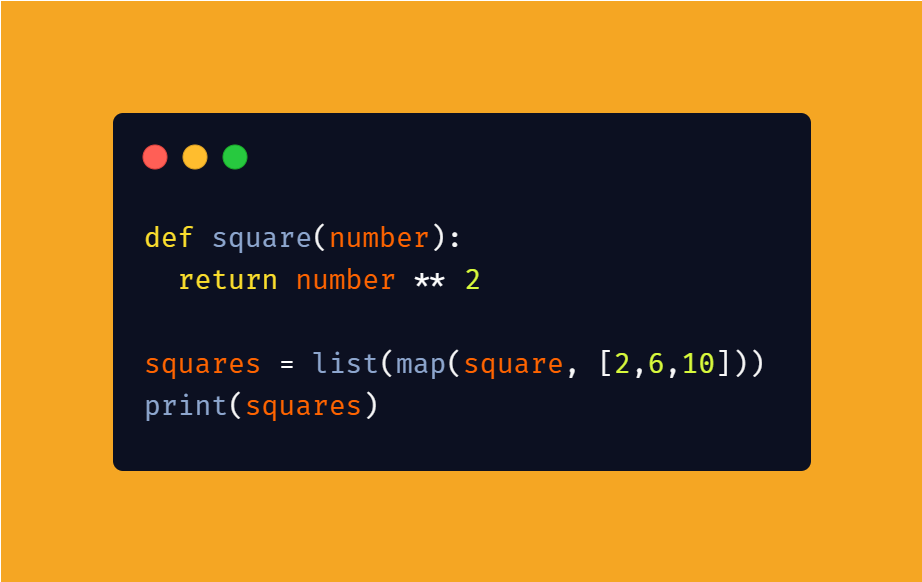Output:

The most common and useful use case of map() function is to receive multiple unknown numbers of input from the user: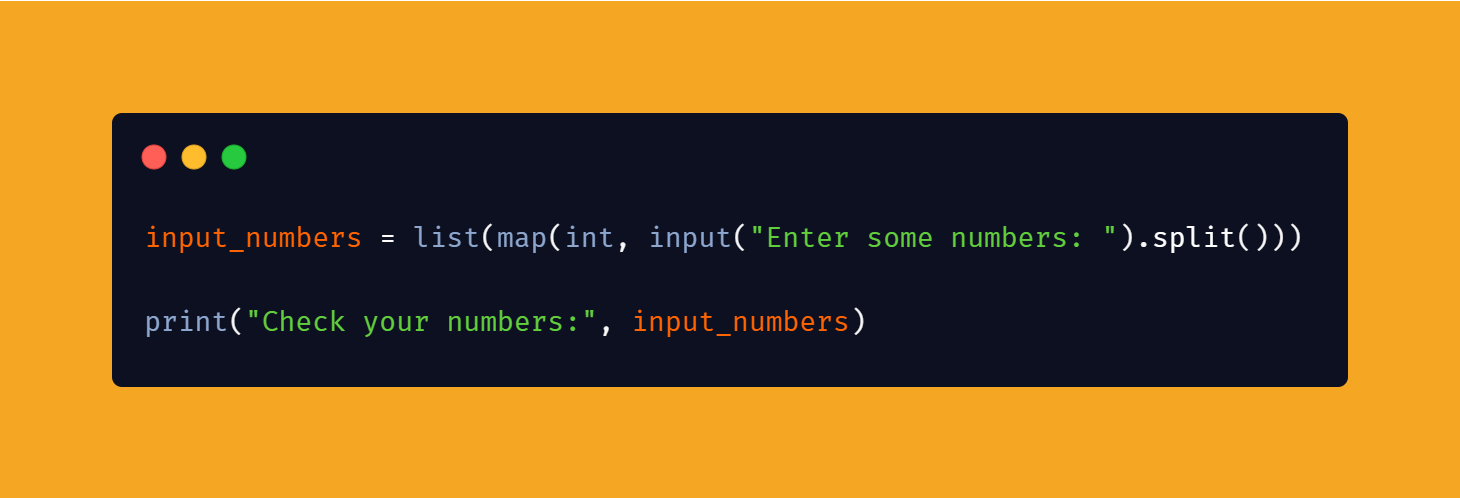Output:

``````Enter some numbers: 2 5 3 7 4
Check your numbers: [2, 5, 3, 7, 4]``````

## 2) filter()

filter() function filters any given iterable based on the specified function.
Consider the following example where the filter() function filters only the items which satisfy the condition of the given only_even() function.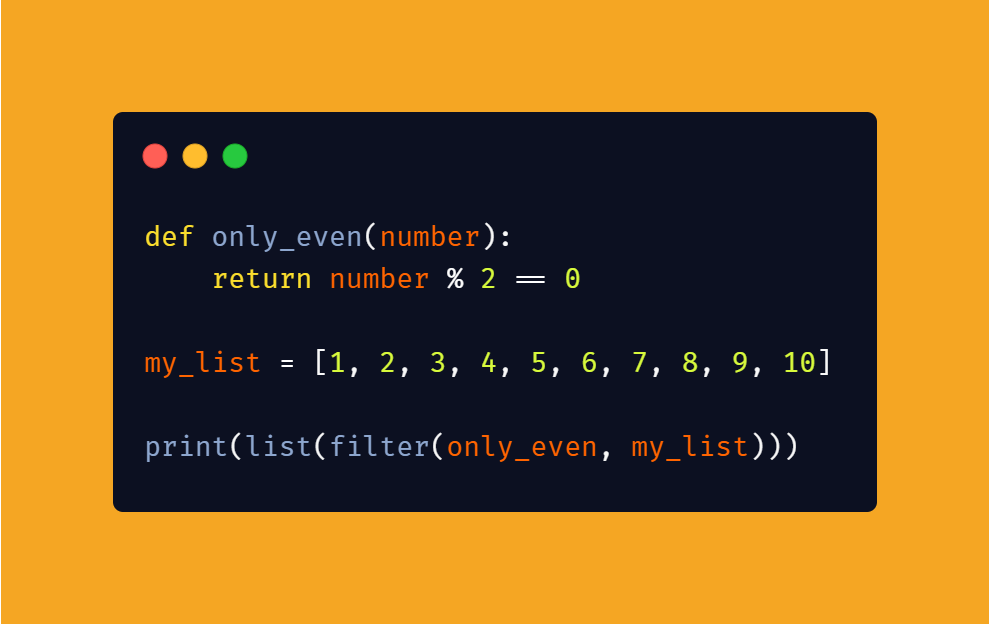Output:

## 3) zip()

When we need to zip two iterables literally, we use the zip() function. We can add as many iterables as we want inside a zip() function. Consider the following example with two iterables: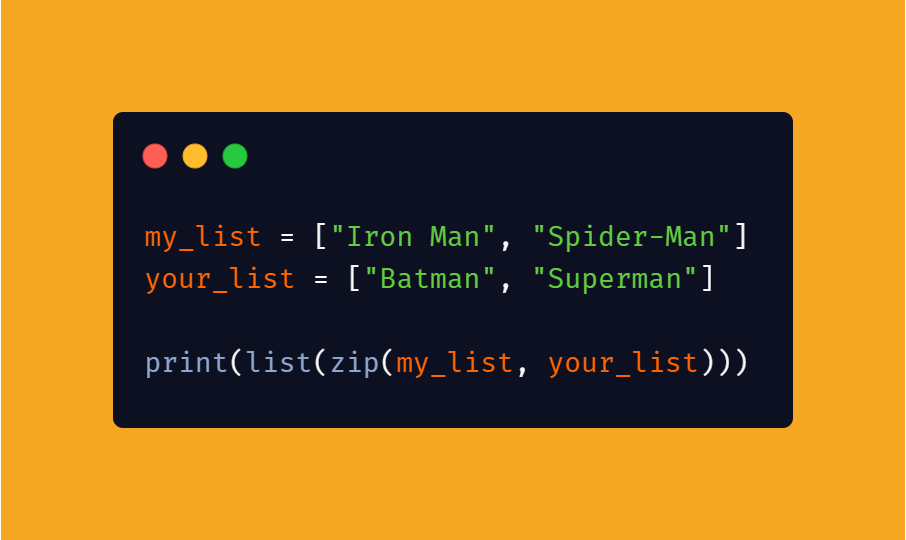Output:

``[('Iron Man', 'Batman'), ('Spider-Man', 'Superman')]``

zip() function binds together the corresponding index items in a tuple and inserts into a list function (as I specified as the list() function).

## 4) reduce()

reduce() function is part of a functools module - a standard python library.
Consider the following example, where the reduce() function accumulates the given list values and provides a final output.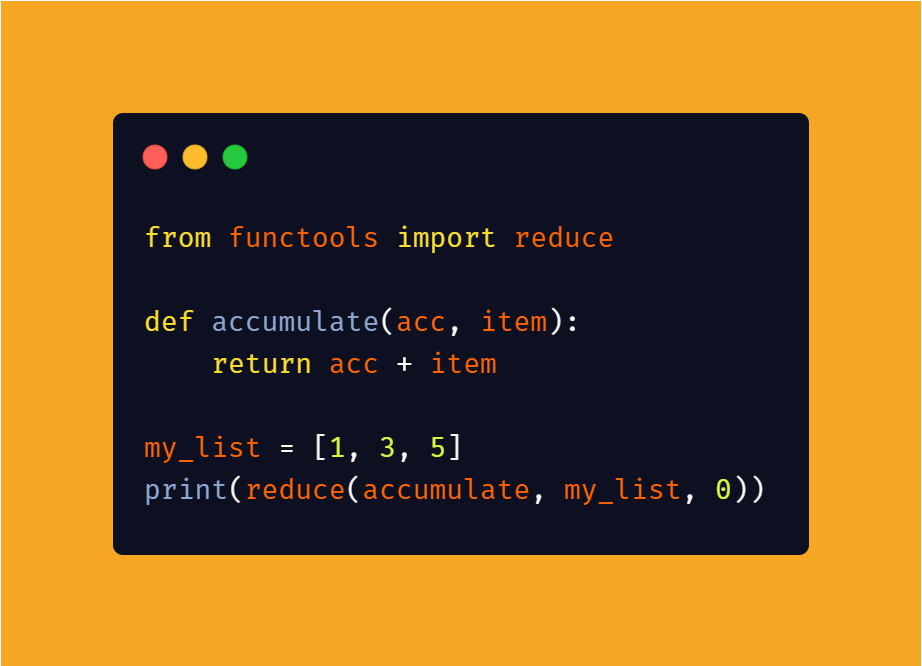Output:

Unlike other functions, the reduce() function accepts three arguments.
From the example, you can see that the function accumulate() accepts two arguments acc and item and returns the sum. Here, the reduce() function passes the last argument 0 as acc at first and the first item from the list, then it accumulates and passes the sum as acc for the next list item and so on until all the list items are accumulated and the result is displayed.

Code along and learn more…

Previously published at https://aswinbarath.github.io/functional-programming-in-python/

Join Hacker Noon

Create your free account to unlock your custom reading experience.